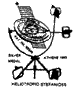Panagiotis Stefanides

ROOT GEOMETRIES STRUCTURING THE ICOSAHEDRON,
THE OTHER BASIC POLYHEDRA AND THE GEOMETRIC FORMS RELATED TO THEM

By

*Eur Ing Panagiotis Chr. Stefanides BSc(Eng)Lon(Hons) MSc(Eng)NTUA CEng MIET
8, Alonion St., Kifissia, Athens, GR-14562

Tel: ++302108011291, E-mail: panamars@otenet.gr

Under  Root Geometries Structuring the Icosahedron, the other Basic Polyhedra and the Geometric Forms Related to Them, we refer to the basic geometric configurations  which, as this theory contemplates, are necessary for  the progressive mode of  formation of the  five polyhedra, via lines, areas and volumes.

The Root Geometries are two configurations of triangles. A Special one, the Quadrature  Scalene Orthogonal  Triangle [Author’s interpretation of  the  Timaeic Most Beautiful Triangle] with sides [T^3], [T^2] and [T^1] in geometric ratio T, which is the square root of the golden ratio[ Φ], and the Isosceles Orthogonal Triangle, with its equal sides [T]. The surface areas of these triangles are taken perpendicular to each other and in such, naturally, defining an X, Y, and Z system of coordinate axes. In so, the coordinates of the first are [0,0,0,], [0,0,T^2], [T,0,0] in the X-Z plane, and  those of the second are [0,0,0,], [T,0,0], [0,T,0] in the X-Y plane. A line from  [0,T,0] to [0,0,T^2],creates  the same Scalene Triangle in the Y, Z plane.

Arctan [T] is the Scalene angle [θ] of the Special Triangle with the property that the product of its small side by its hypotenuse is equal to the square of its bigger side: [T^1]*[T^3] equal [T^2]^2 [Quadrature].

Using a pair of  the  Special Scalene  Triangle, and a pair of a Similar Kind of Triangle [Constituent of the Special] with sides 1,T and T^2 [Kepler's (Magirus) Triangle with sides 1, sqrt(Φ), and Φ] a Tetrahedron [dicta Form 1] is obtained, by appropriately joining the edges of the four  triangles, with coordinates: [0,0,0,], [0,0,T^2], [T,0,0] and [0, 1/T, 1/T^2].

By joining, a line, from point [0,T,0] to point [T,0,0], a Second Tetrahedron [dicta Form 2]  is obtained [ as  a natural  extension of Form 1], with co-ordinates: [0,0,0,], [T,0,0],[0,T,0] and [0,1/T, 1/T^2], having as base, on the X-Y plane, the Isosceles Orthogonal Triangle mentioned above, with coordinates[0,0,0], [T,0,0] and [0,T,0].

Doubling this triangle, in the X-Y plane, a square is obtained of side [T], with coordinates [T,T,0], [T,0,0], [0,0,0],  and [0,T,0].

By connecting a line from point [T,T,0] to point [0,0,T^2] a third Tetrahedron [dicta Form 3] is obtained with coordinates:[T,T,0],[T,0,0], [0,T,0] and [0,0,T^2],

having also as base,  the Isosceles Orthogonal Triangle with same dimensions [mirror image] as  that of [Form 2]. The three Forms are wedged firmly together, leaving no empty space between them. Their volume ratios Form 3: Form 1: Form 2 equal to  [1/6]*[T*T*T^2]: [1/6]*[1*T*T]: [1/6]*[T*T*(1/T^2)] is the golden ratio

[T^2], and the sum of volumes of  Form 1 and  Form 2 equals  to [1/6]*[1*T*T ] + [1/6]*[T*T*(1/T^2)] equals to [1/6]*[T^2+1] equal [1/6]*T^4[SINCE T^4-T^2-1=0],
the volume of Form 3.The volumes of the three Forms sum up to [(2/6)T^4 equal to (1/3)T^4].

Two of the four bases of  Form 3, are symmetrical orthogonal triangles, with coordinates  [T,0,0], [T,T,0], [0,0,T^2] and  [T,T,0], [0,T,0], [0,0,T^2], each of which has an angle [ φ], equal to arctan[T^2].

Two such triangles joined coplanarily and symmetrically along their bigger vertical sides, create one of  the four triangular faces of a great pyramid model with coordinates [T,T,0], [0,0,T^2] and [T,(-T),0].

The Structure of the three Forms bound together [dicta Form 4] with Volume [1/3]*T^4 is one quarter of the volume of the great pyramid model, which has a square base of side 2T, height T^2 and Volume [4/3]*T^4.

Splitting one of  this model’s  triangular face  into two orthogonal  co-planar  triangles to form a parallelogramme [with sides T^1 and T^3], we have constructed  the basic skeleton of  the  Icosahedron, since three such parallgrammes, orthogonal to each other, determine  its  twenty equilateral  bases, by joining adjacent corners in groups of three, by lines.

Similarly, we proceed to the construction of the
dodecahedron,  the  tetrahedron, the octahedron and the cube,  together with their related forms  such as  squares, circles, triangles, circumscribed  circles  to the parallelogrammes of  the polyhedra skeletons, circumscribed spheres  and  logarithmic spirals.

Reversing the whole process, the volumes decompose to the areas of the triangle surfaces structuring them which, in turn, resolve to four line traces harmonically codified  in space.

#### *Ex Hellenic Aerospace Ind., R and D Lead Engineer and Directorate Aircraft Engines’ Manufacturing Engineering Methods’ Superintendent. (c.v.)

Conference: Symmetry Festival 2006 Budapest:
“Golden Root Symmetries of Geometric Forms”

ublication: “Golden Root Symmetries of Geometric Forms” Symmetry: Culture and Science, The journal of the Symmetri0n ,Volume 17, Numbers 1-2, 2006,
pp 97-111.
Award: Archimedes Silver Medal for the Invention of a Solar Locating and Tracking  System, by the Hellenic Society of Research and Inventions, Athens Hilton 4 Feb 1983.
©1986-2011,  Panagiotis Chr. Stefanides, Athens 15/04/2011.